# 机器学习理论总结

2019-01-10

## 1 方差与偏差

$Error = Bias + Variance + Noise$

$\hat f(x)=E_D[f(x;D)]$

$bias(x)=\hat f(x)-y$

$var(x)=E_D[(f(x;D)-\hat f(x))^2]$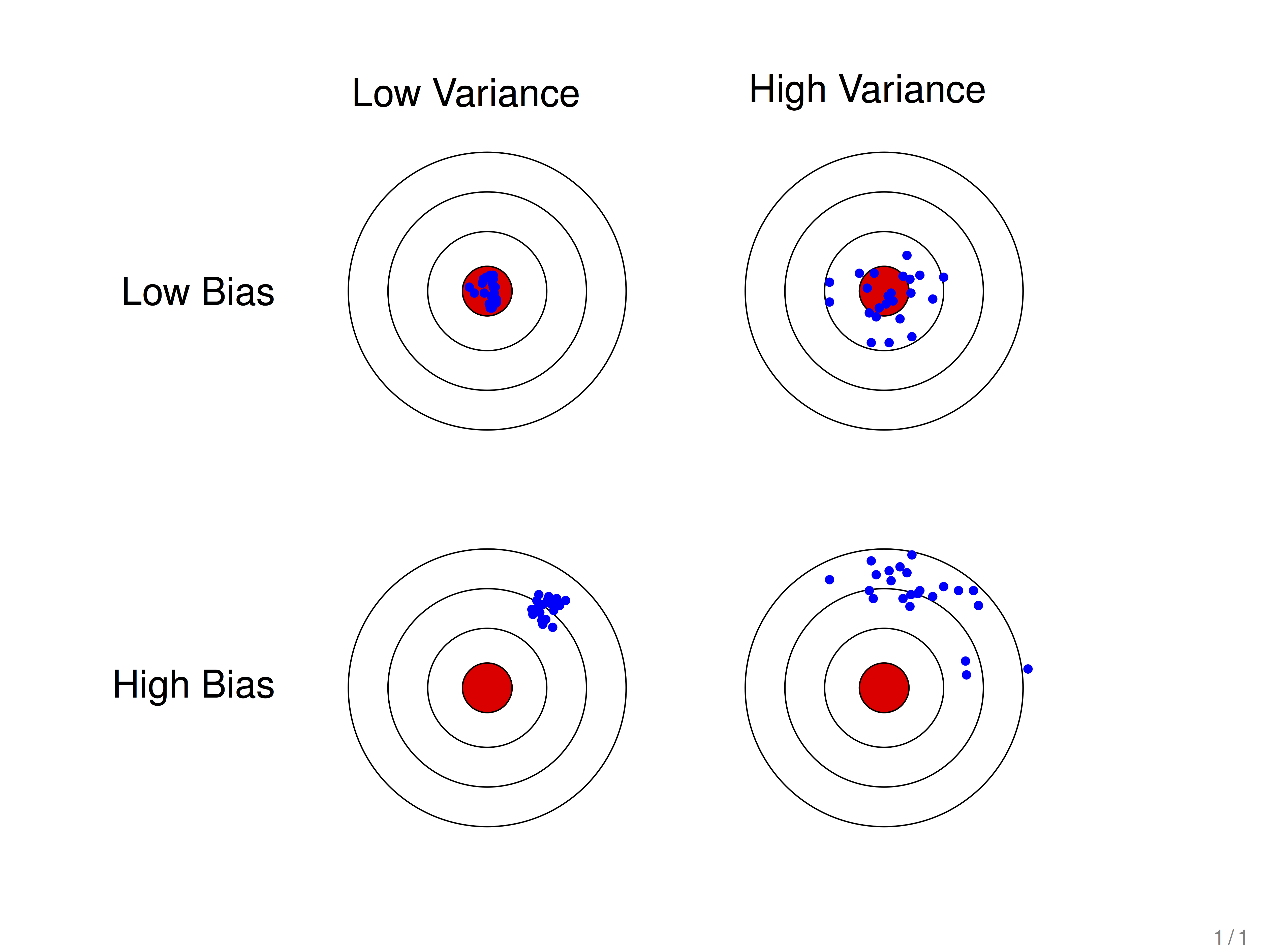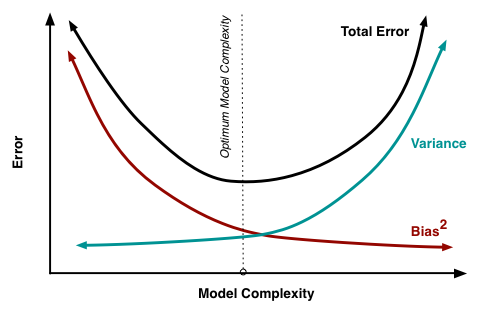## 2 过拟合欠拟合问题

• 高偏差：训练误差大，验证误差大。即欠拟合。
• 高方差：训练误差小，验证误差大。即过拟合。

### 2.1 多项式回归中偏差与方差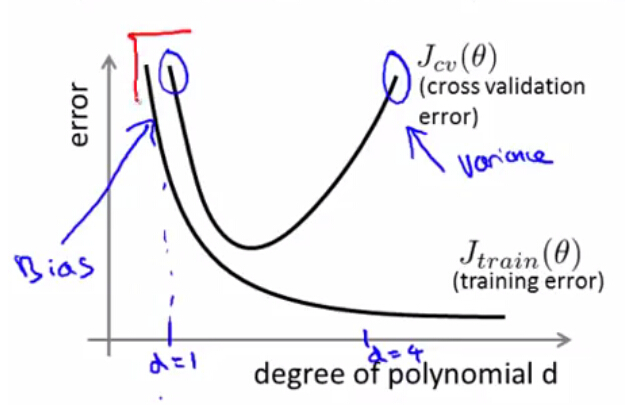### 2.2 正规化过程的偏差与方差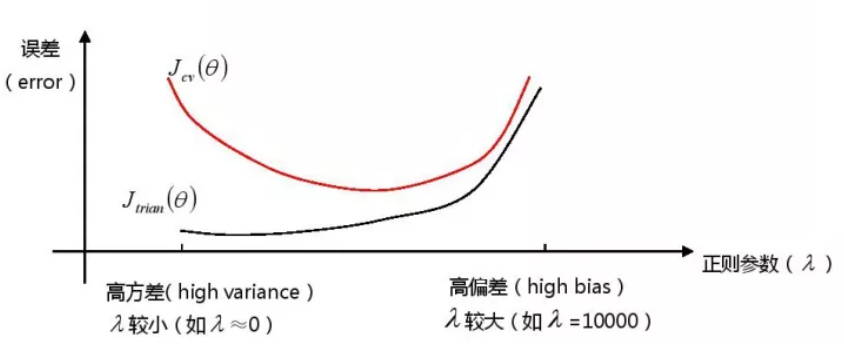### 2.3 样本数目对与偏差方差的影响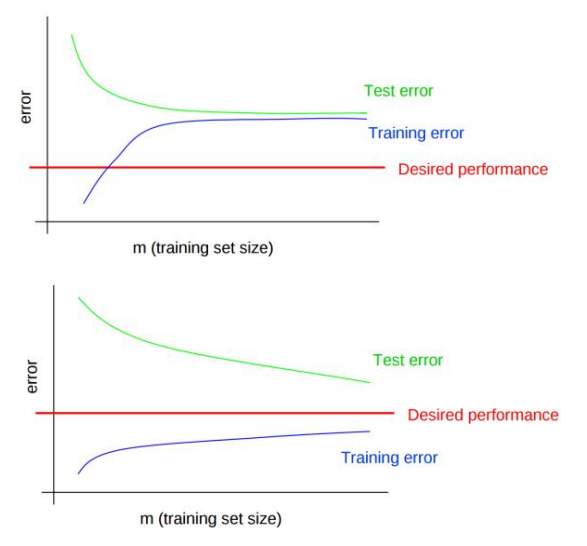• 对于高偏差模型(上图第一个)，训练误差和验证误差很接近，但都处于很大的误差水平之上。此时，无论怎么增加训练集数目，都无法降低误差。
• 对于高方差模型(上图第二个)，训练误差在很低的水平，但是验证误差和训练误差的缺口很大，此时增加训练集样本数目，可以使得验证误差更接近训练误差。即当模型出现高方差问题，可以通过增加样本数目来降低方差。

### 2.4 解决方法

1. 解决欠拟合：
• 增加特征个数$n$
• 增加多项式阶数$d$
• 减小正则项参数值$\lambda$;
• Boosting ,Boosting 往往会有较小的 Bias，比如 Gradient Boosting等。
2. 解决过拟合：
• 减少特征个数$n$
• 减少多项式阶数$d$
• 增加正则项参数值$\lambda$
• 增加训练集样本$m$
• Bagging ,将多个弱学习器Bagging 一下效果会好很多，比如随机森林等。

## 3 L1正则化和L2正则化

L1正则化：是指权值向量$w$中各个元素的绝对值之和，通常表示为$||w||_1$
L2正则化：是指权值向量$w$中各个元素的平方和然后再求平方根（可以看到Ridge回归的L2正则化项有平方符号），通常表示为$||w||_2$

• L1正则化可以产生稀疏权值矩阵，即产生一个稀疏模型，可以用于特征选择（权重为0表示对应的特征没有作用，被丢掉），也可以增强模型可解释性（例如研究影响疾病的因素，只有少数几个非零元素，就可以知道这些对应的因素和疾病相关）
• L2正则化可以防止模型过拟合（overfitting）；一定程度上，L1也可以防止过拟合